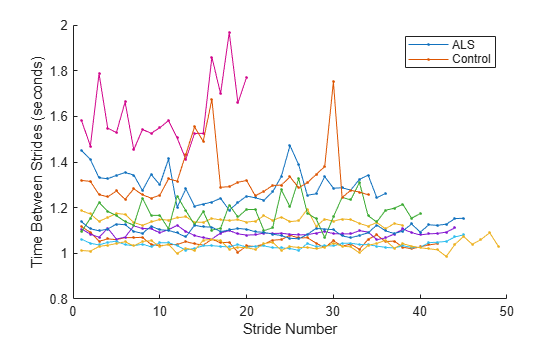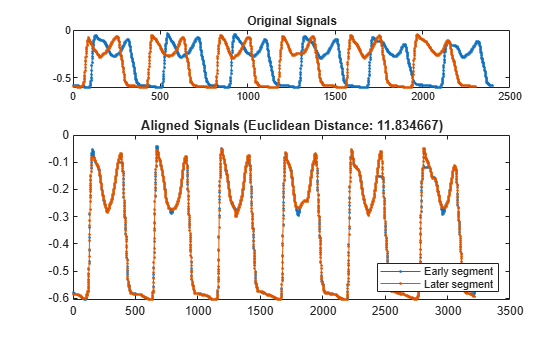Main Content

# Extracting Classification Features from Physiological Signals

This example shows how to use the functions `midcross` and `dtw` to extract features from gait signal data. Gait signals are used to study the walking patterns of patients with neurodegenerative disease. The time between strides has been reported to differ between healthy and sick individuals. `midcross` offers a convenient way to calculate these times. People also change their walking speed over time. `dtw` provides a convenient way to quantitatively compare the shape of gait signals by warping to align them in time. This example uses `midcross` to locate each step in a gait signal and `dtw` to compute distances between gait signal segments. These results are examined as potential features for signal classification. While this example is specific to gait signals, other physiological signals, such as electrocardiogram (ECG) or photoplethysmogram (PPG), can also be analyzed using these functions.

### Measure Inter-Stride Time Intervals

The dataset being analyzed contains force data collected during walking for patients with Amyotrophic Lateral Sclerosis (ALS) and a control group. ALS is a disease made famous by Lou Gehrig, Stephen Hawking, and the 2014 'Ice Bucket Challenge'.

Load and plot the first 30 seconds of gait signal data for one patient.

```helperGaitPlot('als1m'); xlim([0 30])```This dataset represents the force exerted by a foot on a force sensitive resistor. The force is measured in millivolts. Each record is one minute in length and contains separate channels for the left and right foot of a subject. Each step in the dataset is characterized by a sharp change in force as the foot impacts and leaves the ground. Use `midcross` to find these sharp changes for an ALS patient.

Use `midcross` to find and plot the location of each crossing for the left foot of an ALS patient. Chose a tolerance of 25% to ensure that every crossing is detected.

```Fs = 300; gaitSignal = helperGaitImport('als1m'); midcross(gaitSignal(1,:),Fs,'tolerance',25); xlim([0 30]) xlabel('Sample Number') ylabel('mV')````midcross` correctly identifies the crossings. Now use it to calculate the inter-stride times for a group of ten patients. Five patients are control subjects, and five patients have ALS. Use the left foot record for each patient and exclude the first eight crossings to remove transients.

```pnames = helperGaitImport(); for i = 1:10 gaitSignal = helperGaitImport(pnames{i}); IND2 = midcross(gaitSignal(1,:),Fs,'Tolerance',25); IST{i} = diff(IND2(9:2:end)); varIST(i) = var(IST{i}); end```

Plot the inter-stride times.

```figure hold on for i = 1:5 plot(1:length(IST{i}),IST{i},'.-r') plot(1:length(IST{i+5}),IST{i+5},'.-b') end xlabel('Stride Number') ylabel('Time Between Strides (sec)') legend('ALS','Control')```The variance of the inter-stride times is higher overall for the ALS patients.

### Measure Similarity of Walking Patterns

Having quantified the distance between steps, proceed to analyze the shape of the gait signal data independent of these inter-step variations. Compare two segments of the signal using `dtw`. Ideally, one would compare the shape of the gait signal over time as treatment or disease progresses. Here, we compare two segments of the same record, one segment taken early in the recording (`sigsInitialLeft`), and the second towards the end (`sigsFinalLeft`). Each segment contains six steps.

Load the gait signal data segments.

`load PNGaitSegments.mat`

The patient does not walk at the same rate throughout the record. `dtw` provides a measure of the distance between segments by warping them to align them in time. Compare the two segments using `dtw`.

```figure dtw(sigsInitialLeft{1},sigsFinalLeft{1}); legend('Early segment','Later segment','location','southeast')```The two segments are aligned in time. Although the step rate of the patient appears to change over time, as can be seen in the offset of the original signals, `dtw` matches the two segments by allowing samples of either segment to repeat. The distance via `dtw`, along with the variance of the inter-stride times, will be explored as features for a gait signal classifier.

### Construct a Feature Vector to Classify Signals

Suppose you are building a classifier to decide whether or not a patient is healthy based on a gait signals. Investigate the variance of inter-stride times, `feature1`, and the distance via `dtw` between initial and final signal segments, `feature2`, as classification features.

Feature 1 was previously computed using `midcross`.

`feature1 = varIST;`

Extract Feature 2 for the ALS patients and the control group.

```feature2 = zeros(10,1); for i = 1:length(sigsInitialLeft) feature2(i) = dtw(sigsInitialLeft{i},sigsFinalLeft{i}); end```

Plot the features for ALS subjects and control subjects.

```figure plot(feature1(1:5),feature2(1:5),'r*',... feature1(6:10),feature2(6:10),'b+',... 'MarkerSize',10,'LineWidth',1) xlabel('Variance of Inter-Stride Times') ylabel('Distance Between Segments') legend('ALS','Control')```ALS patients seem to have a larger variance in their inter-stride times, but a smaller distance via `dtw` between segments. These features compliment each other and can be explored for use in a classifier such as a Neural Network or Support Vector Machine.

### Conclusions

`midcross` and `dtw` provide a convenient way to compare gait signals and other physiological data which repeat irregularly over time due to different rates of motion or activity. In this example, step times were located using `midcross` and segment distances were computed using `dtw`. These were complimentary measures, as `dtw` removed any time variation that `midcross` distances would measure. As features, these two metrics showed separation between control and ALS patients for this dataset. `midcross` and `dtw` could likewise be used to examine other physiological signals whose shape varies as a function of activity.

### References

 Goldberger, A. L., L. A. N. Amaral, L. Glass, J. M. Hausdorff, P. Ch. Ivanov, R. G. Mark, R. G. Mietus, G. B. Moody, C.-K. Peng, and H. E. Stanley. "PhysioBank, PhysioToolkit, and PhysioNet: Components of a New Research Resource for Complex Physiologic Signals." Circulation. Vol. 101, Number 23, 2000, pp. e215-e200.

## Support

#### Deep Learning for Signal Processing with MATLAB

Download white paper